November 25, 2021

# Class 10 Maths Chapter 5 Arithmetic Progressions

There are many things in nature that follow a certain pattern and not just things there are many situations in life where one can observe a certain pattern like the pattern of increment in salary i.e n the first year one may receive the salary of Rs.20K and next year it may raise to Rs.25K and then next year to Rs.30K and so on. Here you can see that the salary is increasing by Rs.5K every year, so we can say that it is following a certain pattern.

In class 10 maths chapter 5 arithmetic progressions we will learn one such pattern where succeeding terms are obtained by adding a fixed number to the preceding terms. This fairly new topic that you have never studied in previous classes, but don’t worry there is nothing that you can not understand with the right effort.

## Class 10 Maths Chapter 5 Arithmetic Progressions

If you want to learn something then the correct approach is to start the very basics of it and then proceed to the advanced topics. This is what we are going to do with this AP (arithmetic progressions). We will first learn the basic terminology that is used in this chapter and then see the formulas and some examples.

• What is progression? In Lehman language, a sequence of numbers that follows a certain pattern is called progression.
• What is Arithmetic progression (AP)? The list of numbers in which each term is achieved by adding a fixed number to the preceding term (except the first) is called an arithmetic progression or AP.
• Term: The word ‘term’ for class 10 maths chapter 5 arithmetic progressions is given to each number in an AP.
• Common difference (d) = the fixed number in the above given AP definition is called the common difference and is denoted by ‘d’. Keep in mind that the common difference can be anything i.e a negative number, or a positive number, or even zero.
• The general form of an AP: When an AP is represented in a particular fashion where ‘a’ is the first term and ‘d’ is a common difference, then it is called the general form of an AP. e.g a, a+d, a+2d, a+3d…
• Finite AP: When there is a finite number of terms in an AP, then it is called a finite AP.
• Infinite AP: For AP’s having infinite terms or we can say that they do not have the last term is called infinite AP’s.

### Class 10 Maths Chapter 5 Arithmetic Progressions Explanation

Since the terminologies are clear to us now, we can move to learn the main concepts of this chapter, which is finding nth terms and sum of nth terms of an AP.

# nth term of an AP

From the example of salary that we took at the beginning of this article, we can easily determine what will be the salary after 2, 3 or even 10 to 20 years, but what if we want to know the salary after 60 or 70 years, then calculating it with the conventional methods will be a daunting task. This is why we have the formula for calculating the nth term of an AP. Here, ‘n’ can be any value.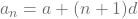, here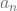= value of the term that we want to find (sometimes also denoted by ‘l’), a = first term of AP, n = the term in Ap that we want to find, d = command difference.

Students may get confused betweenand ‘n’. So, to clear this confusion, let’s understand it with an example.

Suppose that there is an AP with 6 terms i.e 10, 20, 30, 40, 50, 60 (assume that we only know the starting 2 terms). Let’s assume that we are to find the 4th term and its value in this AP. So, here n = 4 and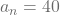.

# Sum of first n terms of an AP

We know what an AP is, we also know how to get the value nth terms and its value. In this section, we will learn how to find the sum of the first nth terms of an AP.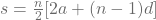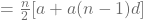since we know that, therefore,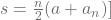Also, we saw thatcan also be denoted as ‘l’, therefore,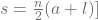Using the above-given formulas we can find out the sum of nth terms of an AP.

the sum of first n positive integers is given by: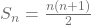Here is an extra knowledge for you. If a, b, then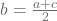and b are called the arithmetic
mean of a and c. This is an advanced concept that you will learn in future classes, but for those who are going to prepare for competitive exams, this is a topic that you can practice in class 10th with refreshers like RD Sharma and RS Agarwal. Remember that performing in the board exam should be your priority, go for this topic only if you have spare time and once you complete the syllabus-related topics first.

Class 10 maths chapter 5 arithmetic progressions is one of the toughest chapters in class 10th because this is a fairly new concept to students. One needs to have absolute attention while practicing AP, make sure to be 100% dedicated while learning this chapter.